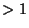## Fundamental Theorem of Algebra

Every Polynomial equation having Complex Coefficients and degreehas at least one Complex Root. This theorem was first proven by Gauß.It is equivalent to the statement that a Polynomialof degreehasvalues of(some of them possibly degenerate) for which. An example of a Polynomial with a single Root of multiplicityis, which hasas a Root of multiplicity 2.